# 用 PyTorch 做 神经转换 (Neural Transfer)

## 介绍

### 题中的神经描述的是什么?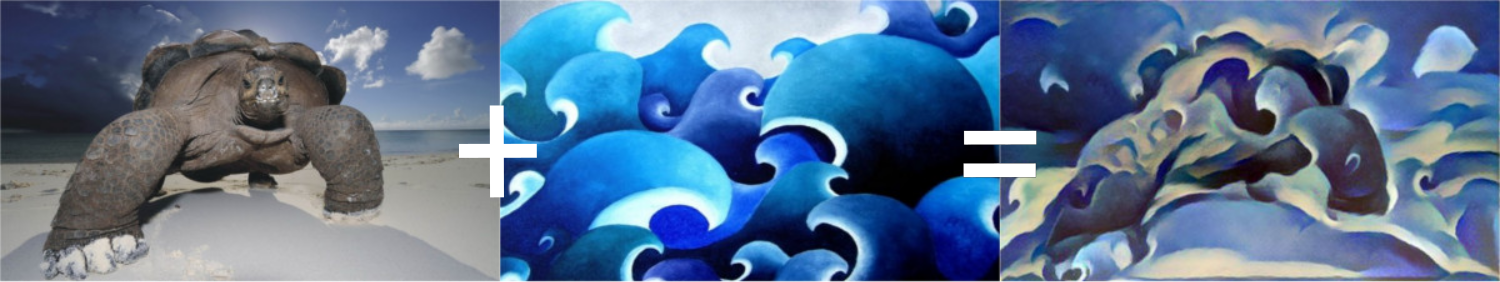### 它是如何工作的?

#### 好吧, 它具体是怎么工作的?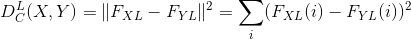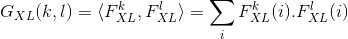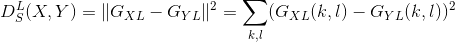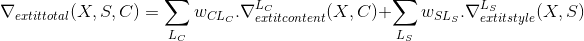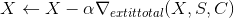## PyTorch 实现

### 包

• torch, torch.nn, numpy (indispensables packages for neural networks with PyTorch)
• torch.autograd.Variable (dynamic computation of the gradient wrt a variable)
• torch.optim (efficient gradient descents)
• PIL, PIL.Image, matplotlib.pyplot (load and display images)
• torchvision.transforms (treat PIL images and transform into torch tensors)
• torchvision.models (train or load pre-trained models)
• copy (to deep copy the models; system package)
from __future__ import print_function

import torch
import torch.nn as nn
import torch.optim as optim

from PIL import Image
import matplotlib.pyplot as plt

import torchvision.transforms as transforms
import torchvision.models as models

import copy


### Cuda

use_cuda = torch.cuda.is_available()
dtype = torch.cuda.FloatTensor if use_cuda else torch.FloatTensor


### 读取图像

# 想要的输出图像尺寸
imsize = 512 if use_cuda else 128  # 如果没有 GPU 则使用小尺寸

transforms.Scale(imsize),  # 缩放图像
transforms.ToTensor()])  # 将其转化为 torch 张量

image = Image.open(image_name)
# 由于神经网络输入的需要, 添加 batch 的维度
image = image.unsqueeze(0)
return image

assert style_img.size() == content_img.size(), \
"we need to import style and content images of the same size"


### 显示图像

unloader = transforms.ToPILImage()  # 转回 PIL 图像

plt.ion()

def imshow(tensor, title=None):
image = tensor.clone().cpu()  # 克隆是为了不改变它
image = image.view(3, imsize, imsize)  # 移除 batch 维度
plt.imshow(image)
if title is not None:
plt.title(title)
plt.pause(0.001) # 暂停一会, 让绘图更新

plt.figure()
imshow(style_img.data, title='Style Image')

plt.figure()
imshow(content_img.data, title='Content Image')


### 内容损失

class ContentLoss(nn.Module):

def __init__(self, target, weight):
super(ContentLoss, self).__init__()
# 我们会从所使用的树中“分离”目标内容
self.target = target.detach() * weight
# 动态地计算梯度: 它是个状态值, 不是变量.
# 否则评价指标的前向方法会抛出错误.
self.weight = weight
self.criterion = nn.MSELoss()

def forward(self, input):
self.loss = self.criterion(input * self.weight, self.target)
self.output = input
return self.output

def backward(self, retain_graph=True):
self.loss.backward(retain_graph=retain_graph)
return self.loss


### 风格损失

class GramMatrix(nn.Module):

def forward(self, input):
a, b, c, d = input.size()  # a=batch size(=1)
# b= 特征映射的数量
# (c,d)= 一个特征映射的维度 (N=c*d)

features = input.view(a * b, c * d)  # 将 F_XL 转换为 \hat F_XL

G = torch.mm(features, features.t())  # 计算克产物 (gram product)

# 我们用除以每个特征映射元素数量的方法
# 标准化克矩阵 (gram matrix) 的值
return G.div(a * b * c * d)


class StyleLoss(nn.Module):

def __init__(self, target, weight):
super(StyleLoss, self).__init__()
self.target = target.detach() * weight
self.weight = weight
self.gram = GramMatrix()
self.criterion = nn.MSELoss()

def forward(self, input):
self.output = input.clone()
self.G = self.gram(input)
self.G.mul_(self.weight)
self.loss = self.criterion(self.G, self.target)
return self.output

def backward(self, retain_graph=True):
self.loss.backward(retain_graph=retain_graph)
return self.loss


### 读取神经网络

PyTorch对 VGG 的实现模块分为两个子 Sequential 模块: features (包括卷积和池化层) 和 classifier (包括全连接层). 我们只对 features 感兴趣:

cnn = models.vgg19(pretrained=True).features

# 可能的话将它移到 GPU 上:
if use_cuda:
cnn = cnn.cuda()


Sequential (顺序) 模块包含一个子模块的列表. 比如, vgg19.features 包含一个以正确深度排列的序列 (Conv2d, ReLU, Maxpool2d, Conv2d, ReLU…), 就如我们在 Content loss 部分讲到的, 我们想要把我们的风格和内容损失模块以想要的深度作为 ‘透明层’ 加入到 我们的网络中. 为了这样, 我们建立了一个新的 Sequential (顺序) 模块, 在其中我们把 vgg19 和我们的损失模块以正确的顺序加入:

# 希望计算风格/内容损失的层 :
content_layers_default = ['conv_4']
style_layers_default = ['conv_1', 'conv_2', 'conv_3', 'conv_4', 'conv_5']

def get_style_model_and_losses(cnn, style_img, content_img,
style_weight=1000, content_weight=1,
content_layers=content_layers_default,
style_layers=style_layers_default):
cnn = copy.deepcopy(cnn)

# 仅为了有一个可迭代的列表 内容/风格 损失
content_losses = []
style_losses = []

model = nn.Sequential()  # 新建的 Sequential 网络模块
gram = GramMatrix()  # 我们需要一个克模块 (gram module) 来计算风格目标

# 可能的话将这些模块移到 GPU 上:
if use_cuda:
model = model.cuda()
gram = gram.cuda()

i = 1
for layer in list(cnn):
if isinstance(layer, nn.Conv2d):
name = "conv_" + str(i)

if name in content_layers:
# 加内容损失:
target = model(content_img).clone()
content_loss = ContentLoss(target, content_weight)
content_losses.append(content_loss)

if name in style_layers:
# 加风格损失:
target_feature = model(style_img).clone()
target_feature_gram = gram(target_feature)
style_loss = StyleLoss(target_feature_gram, style_weight)
style_losses.append(style_loss)

if isinstance(layer, nn.ReLU):
name = "relu_" + str(i)

if name in content_layers:
# 加内容损失:
target = model(content_img).clone()
content_loss = ContentLoss(target, content_weight)
content_losses.append(content_loss)

if name in style_layers:
# 加风格损失:
target_feature = model(style_img).clone()
target_feature_gram = gram(target_feature)
style_loss = StyleLoss(target_feature_gram, style_weight)
style_losses.append(style_loss)

i += 1

if isinstance(layer, nn.MaxPool2d):
name = "pool_" + str(i)

return model, style_losses, content_losses


# avgpool = nn.AvgPool2d(kernel_size=layer.kernel_size,


### 输入图像

input_img = content_img.clone()
# 如果你想用白噪声做输入, 请取消下面的注释行:
# input_img = Variable(torch.randn(content_img.data.size())).type(dtype)

# 在绘图中加入原始的输入图像:
plt.figure()
imshow(input_img.data, title='Input Image')


### 梯度下降

def get_input_param_optimizer(input_img):
# 这行显示了输入是一个需要梯度计算的参数
input_param = nn.Parameter(input_img.data)
optimizer = optim.LBFGS([input_param])
return input_param, optimizer


def run_style_transfer(cnn, content_img, style_img, input_img, num_steps=300,
style_weight=1000, content_weight=1):
"""Run the style transfer."""
print('Building the style transfer model..')
model, style_losses, content_losses = get_style_model_and_losses(cnn,
style_img, content_img, style_weight, content_weight)
input_param, optimizer = get_input_param_optimizer(input_img)

print('Optimizing..')
run = 
while run <= num_steps:

def closure():
# 校正更新后的输入图像值
input_param.data.clamp_(0, 1)

model(input_param)
style_score = 0
content_score = 0

for sl in style_losses:
style_score += sl.backward()
for cl in content_losses:
content_score += cl.backward()

run += 1
if run % 50 == 0:
print("run {}:".format(run))
print('Style Loss : {:4f} Content Loss: {:4f}'.format(
style_score.data, content_score.data))
print()

return style_score + content_score

optimizer.step(closure)

# 最后一次的校正...
input_param.data.clamp_(0, 1)

return input_param.data


output = run_style_transfer(cnn, content_img, style_img, input_img)

plt.figure()
imshow(output, title='Output Image')

# sphinx_gallery_thumbnail_number = 4
plt.ioff()
plt.show()